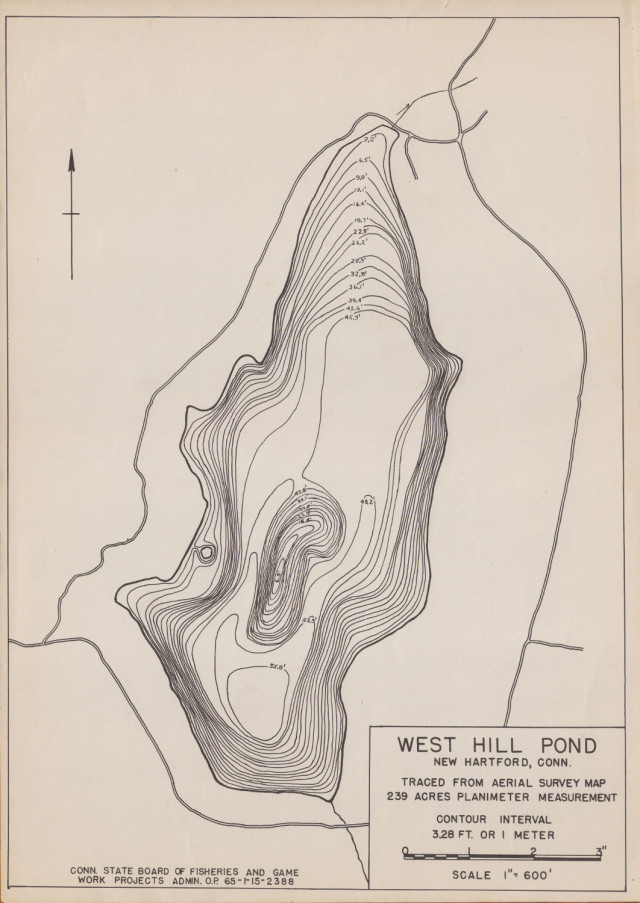# Volume of West Hill Pond

Since I have a bathymetric map of West Hill Pond, I thought it might be interesting to calculate the volume of the pond. The formula for calculating the volume of the frustum of a pyramid is$V = \frac{1}{3} h \left( A_1 + A_2 + \sqrt{A_1 A_2} \right)$ where$h$ is the height of the frustum,$A_1$ is the base area of the top of the frustum, and$A_2$ is the base area of the bottom of the frustum.1 This formula can be used with bathymetric contours to approximate the volume of a water body.2 Applying the formula to the bathymetric map of West Hill Pond results in a calculated volume of 9.3 × 106 m3, or 2.5 billion gallons.Also available is the bathymetric contour shapefile used.

This entry was posted in and tagged , , . Bookmark the permalink.